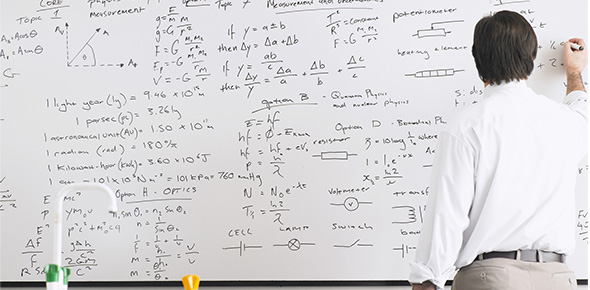# Balancing Equations Quiz 2

5 Questions | Attempts: 349
ShareSettingsUsing your newly-found equation balancing knowledge, balance the following equations and label each equation type. [S]=synthesis [D]=decomposition [SR]= single replacement [DR]=double replacement [CC]=complete combustion [IC]=incomplete combustion

• 1.
What type of reaction is this? ____ Br2 + ____ LiF -->  ____ LiBr + ____ F2
• A.

Synthesis

• B.

Decomposition

• C.

Single replacement

• D.

Double replacement

• 2.
What are the correct coefficients for the following equation? ____ Br2 + ____ LiF -->  ____ LiBr + ____ F2
• A.

2, 4, 4, 2

• B.

1, 2, 3, 1

• C.

1, 2, 2, 1

• 3.
____ Ni(OH)3 --> ____ Ni2O3 + ____ H2OWhen the above equation is balanced, what is the coefficient for nickle (III) oxide?
• A.

2

• B.

1

• C.

3

• 4.
____ K2SO3 + ____ Mn(OH)2 --> ____ KOH + ____ MnSO3 What kind of reaction does the above equation represent?
• A.

Synthesis

• B.

Decomposition

• C.

Single replacement

• D.

Double replacement

• 5.
Complete the following:Mrs. Pippins reminds me of_____________
• A.

"The Grinch" because we have 70 a question semester exam!

• B.

"the best chemistry teacher I have this year."

## Related TopicsBack to top
×

Wait!
Here's an interesting quiz for you.